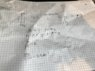# Buouyancy force and Archimedes' principle

lin1430
How much more will the volume of a fishingboat go under water, if I load the boat with 3.0m^3 fish with the density 0.90kg/dm^3?

Fish : 3.0m^3
Density of fish: 0.90kg/dm^3?

## Homework Equations

Archimedes principle: density * volume * g[/B]

## The Attempt at a Solution

Tried setting upward force = downwards force (mg)

substituting m for ro*V[/B]

changing V to A*h, then finding h. But it is obviously wrong.

Homework Helper
Gold Member
Ahoihoi @physicsforum!

Unfortunately it's not possible to comprehend your attempt to solve the problem. Please try to write down all of your steps, explaining your used symbols.

The principle of Archimedes states that the buoyancy force acting on a body corresponds to the weight (force) of the displaced fluid. My first guess, after reading your post, would be that you mixed up the density of the water and the fish...

lin1430
This was my attempt

#### Attachments

•image1.jpeg
87.1 KB · Views: 246
Homework Helper
Gold Member
This was my attempt

I think it would be easier to calculate the additionally displaced volume of water, when the fish is loaded on the boat. You can calculate that with the equation below your drawing, all you need is the values for the densities of fish and water and the volume of fisch you want to load. The data of the fish is provided as you wrote in your first post. Do you have any density given for the water? If not, I propose to use 1 kg/dm^3. But with these three values you are able to calculate the displaced volume by the weight of the fish. I don't know if this is already the demanded answer or if you have to calculate how much the boat will immerge (if the geometry of the boat is given).

•lin1430
lin1430
I think it would be easier to calculate the additionally displaced volume of water, when the fish is loaded on the boat. You can calculate that with the equation below your drawing, all you need is the values for the densities of fish and water and the volume of fisch you want to load. The data of the fish is provided as you wrote in your first post. Do you have any density given for the water? If not, I propose to use 1 kg/dm^3. But with these three values you are able to calculate the displaced volume by the weight of the fish. I don't know if this is already the demanded answer or if you have to calculate how much the boat will immerge (if the geometry of the boat is given).

Sounds good. The density of water is to be 1kg/dm^3. I do not have to calculate how much the boat wil immerge, just how much additional change will happen. But how would I proceed to calculate the displaced volume by the equation under my drawing?

Homework Helper
Gold Member
Sounds good. The density of water is to be 1kg/dm^3. I do not have to calculate how much the boat wil immerge, just how much additional change will happen. But how would I proceed to calculate the displaced volume by the equation under my drawing?

As you wrote in your first post, balance of forces must be fulfilled after loading the fish. The additional weight of the fish must be compensated by additional displaced water. Just equal the mass of the loaded fish (##\rho_FV_F##) and the mass of the displaced water (##\rho_WV_W##); the gravitational acceleration(s) ##g## cancels each other on both sides of the equation. You stick with one unknown (##V_W##) for which you have one equation to solve for it.